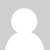### watt calculation with 120V AC-AC adapter

Hi everyone,

First I must admit that I have only basic knowledge of electricity and it is why i'm asking this questions here.

So here is what I understand: V * A = Watts

So I have an emontx v3.4 which is powered by an AC-AC power supply hooked on a 120V outlet.

I don't have any problems monitoring wattage from CTs data hooked on a 120V wire. My question is I'm trying to also monitor 240V wires and since the voltage information gathered from the emontx is from the CA-CA adapter which is reporting 120V, will the wattage calculation corrupted and report only half of what is supposed to show?### Re: watt calculation with 120V AC-AC adapter

Ok, I informed myself a bit more about my home setup. The 240V is actually the combination of the 2 feeds coming from the main in 2 phases. So how should I monitor the 240V appliance with the emontx? Should I have a clamp for the 2 120V hot wires, or will the amp reading be the same on both hands? If so I'll still have to multiply the watt reading by 2.

### Re: watt calculation with 120V AC-AC adapter

You need the article on using the emonTx in the USA, which I haven't written yet! (But there's hope - I have an outline scope drafted.)

Basically, you need to treat one wire out of the three as your reference, and measure the voltage to and the current in the other two wires. Then the total power is sum of the two individual powers. But you're thinking "How do I measure two voltages?" Unfortunately you can't since the emonTx has only one voltage input, but I'm led to believe that the balance between the two 120 V legs is good, so you can assume the same voltage for both. So you measure the voltage on one 120 V leg to neutral, put a CT on both legs. You calculate the two powers separately, with your sketch set up to use the same voltage reference for both power calculations. The current in the two legs will definitely not be the same unless you only have 240 V loads. For those, you measure the current twice but you only measure half the voltage, so the power sum comes right in the end. (You might get equal currents if your 120 V loads are exactly balanced across both legs, but in practice that's unlikely.)### Re: watt calculation with 120V AC-AC adapter

So to make this simple, I have to put 1 CT on each of the hot wire. The voltage calculation is done by the emontx using the AC-AC adapter (~120V), so I simply add the value gathered by the 2 and this would give me the total watt for the 240V breaker/device.

Did I get this right?

### Re: watt calculation with 120V AC-AC adapter

That sounds right.
[Edit - I forgot to mention that the two CT's should face in opposite directions.]